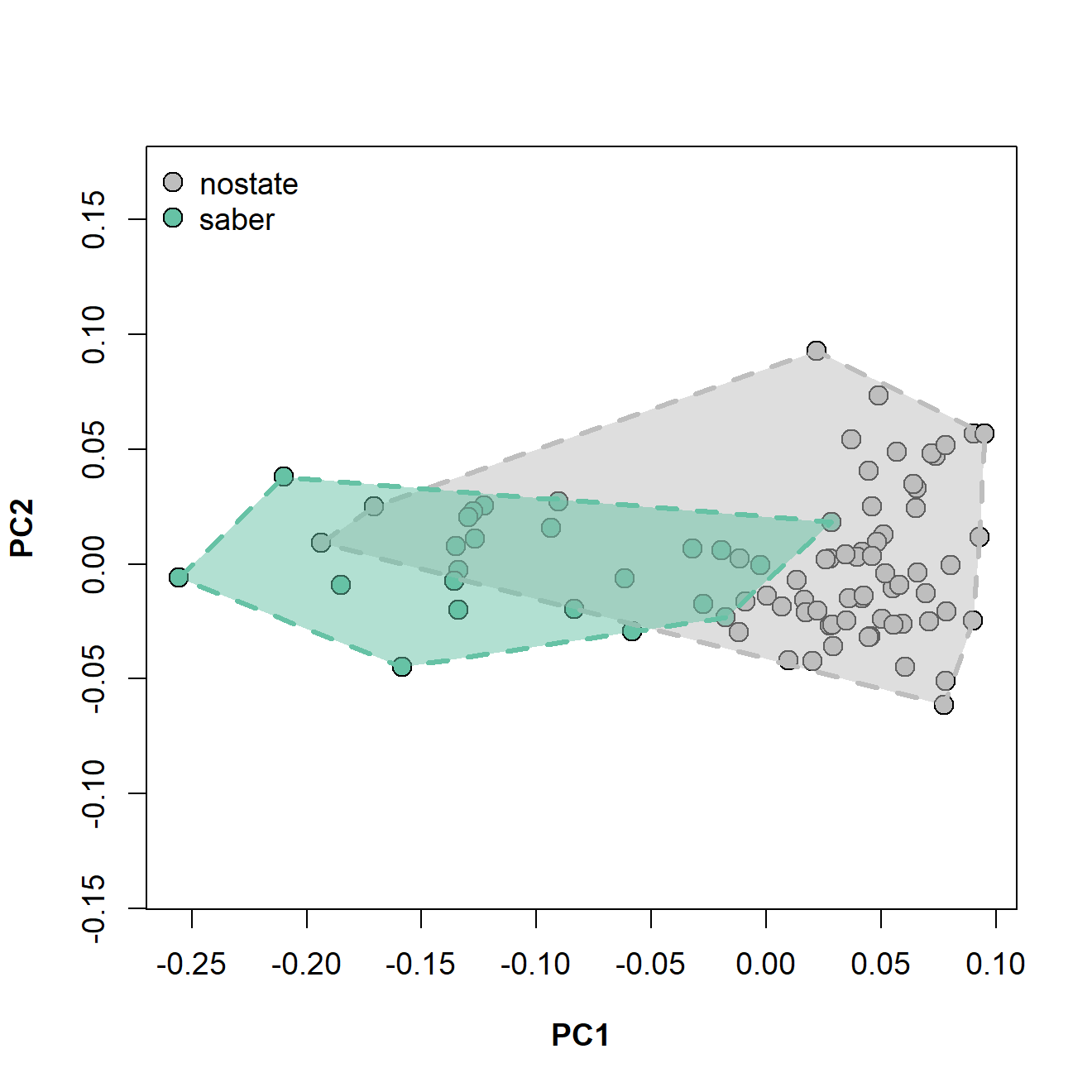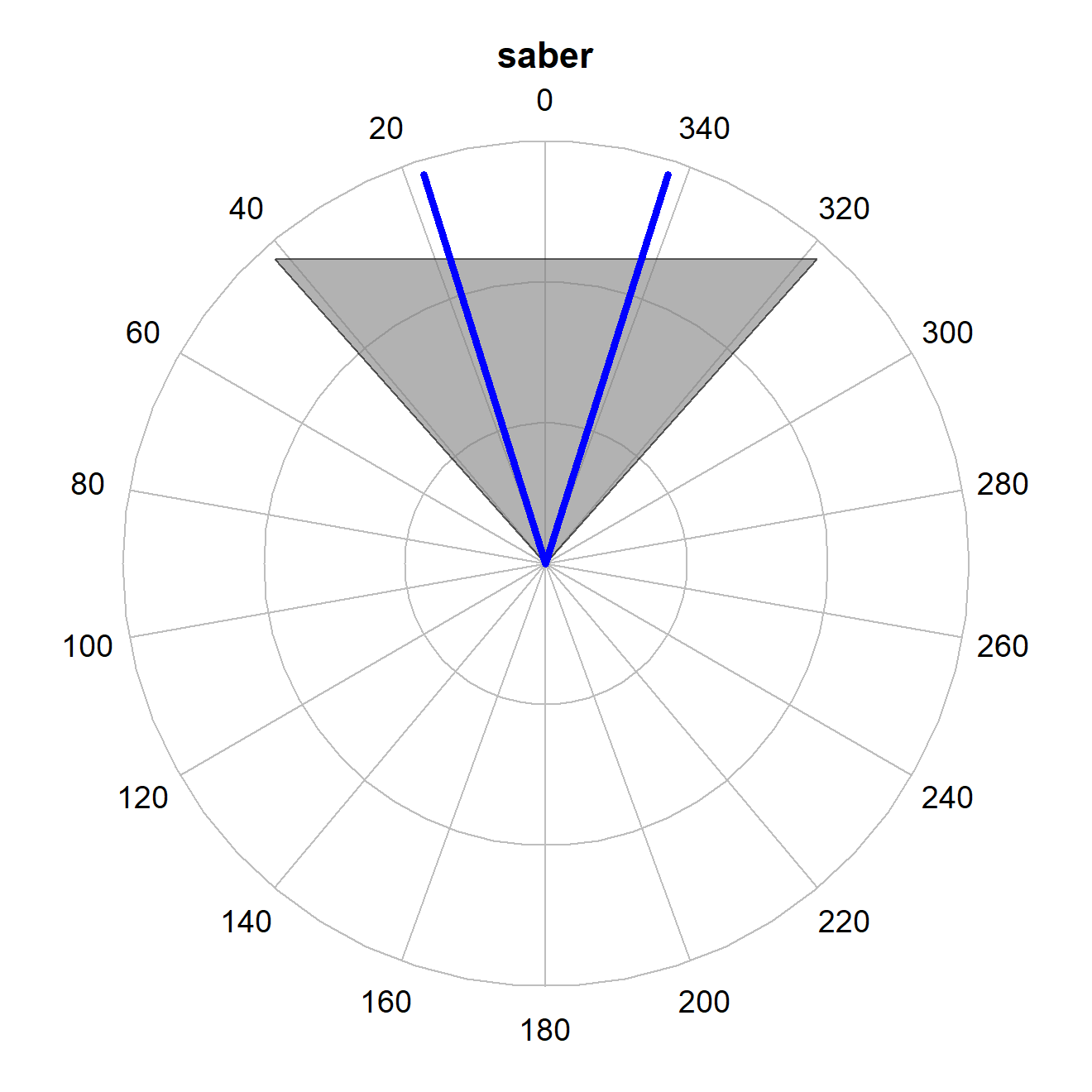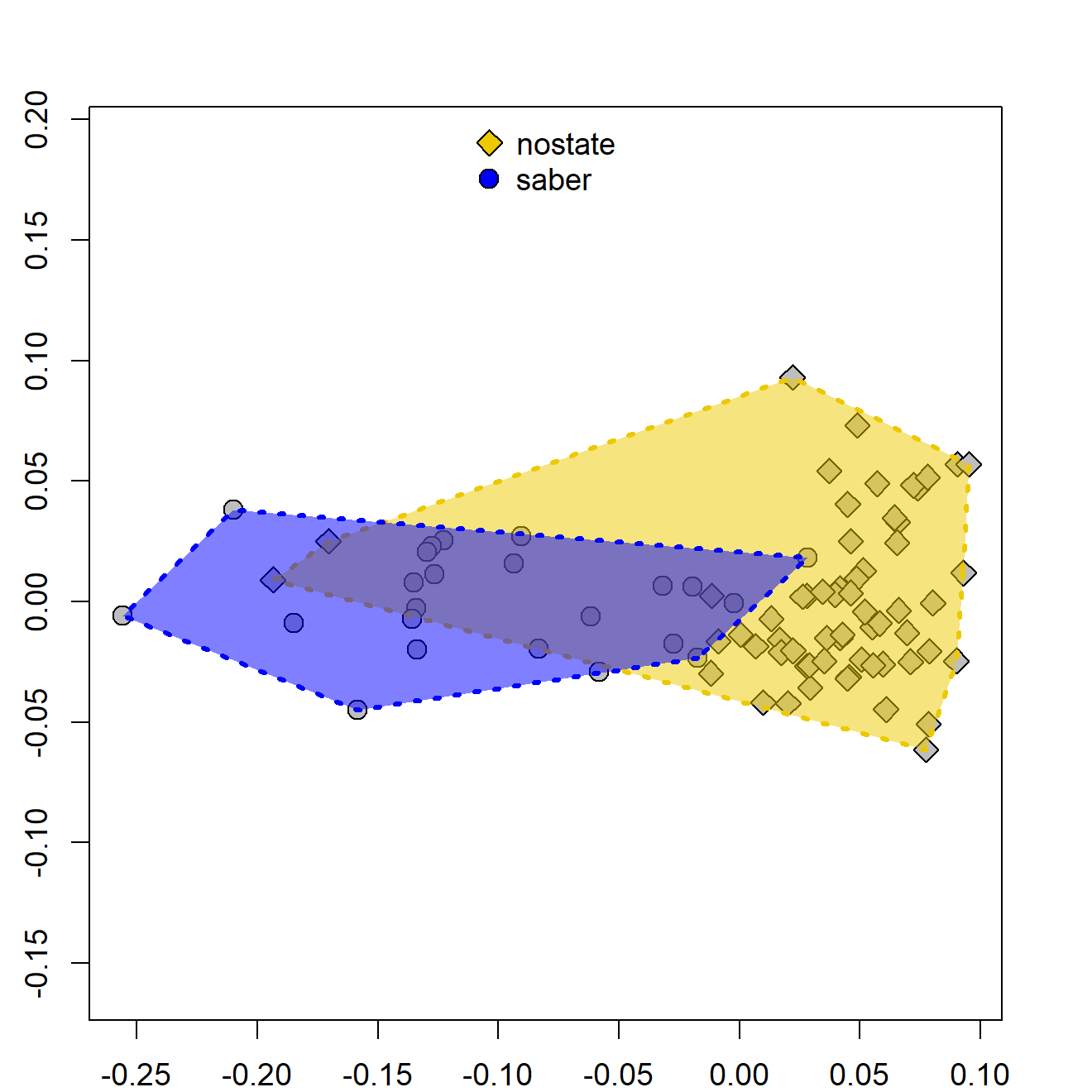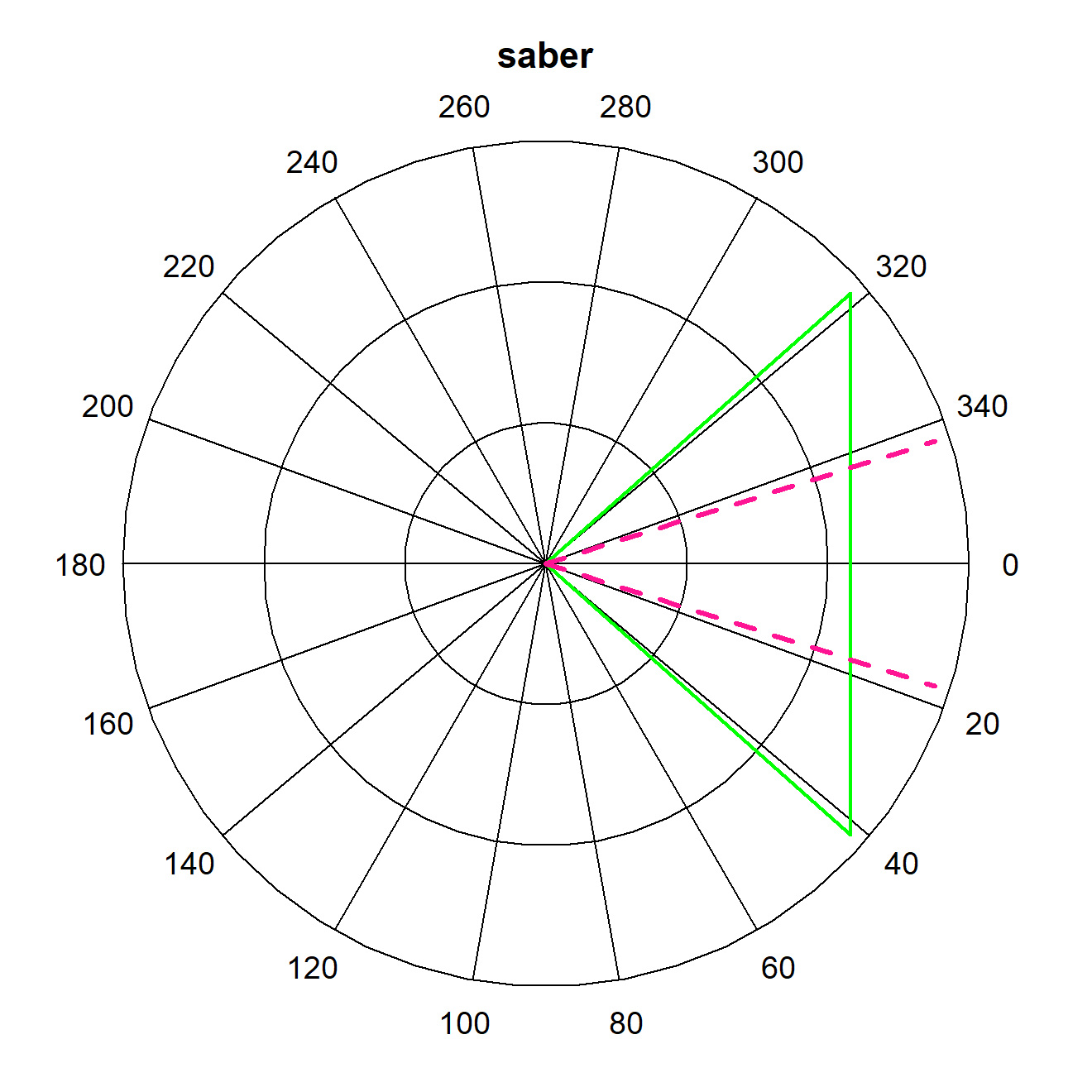# Plotting tools

## plotting RRphylo rates

Evolutionary rates computed by RRphylo for all the tree branches belonging to a specific clade can be visualized by means of plotRates. The function takes as objects the result of RRphylo and the focal node number (located on the tree returned by RRphylo) and returns two customized functions. The function plotHist plots the histograms of rates (in ln absolute values) computed for the focal clade compared to rates computed for the rest of the tree. The plot is completely customizable by providing lists of hist.args, being all the arguments usually passed to the function hist, and legend.args, usually passed to the function legend. The function plotLollipop returns a lollipop plot (see documentation for lollipoPlot function in RRphylo package) of rate values for each branch in the clade. As well as plotHist, this is customizable by indicating lists of lollipop.args for the lollipops and line.args for the vertical line representing the mean rate for the entire tree. Additionally, plotLollipop returns the vector of evolutionary rates for each branch within the focal clade, sorted in the same order they are plotted, that is from the largest to the smallest value. In this way, the user could ideally set different graphical parameters for each species/node in the vector (see the example below).

### load the RRphylo example dataset including Cetaceans tree and ln body masses
data("DataCetaceans")
DataCetaceans$treecet->treecet DataCetaceans$masscet->masscet

# Perform RRphylo
RRphylo(treecet,masscet)->RRcet

# Visualize evolutionary rates computed for the clade including extant Mysticetes
plotRates(RRcet,node=131)->pRates
# Using default options
par(mar=c(3,3,1,1),mgp=c(1.7,0.5,0))
pRates$plotHist() par(mar=c(3,12,1,1),mgp=c(1.7,0.5,0)) pRates$plotLollipop()->pLol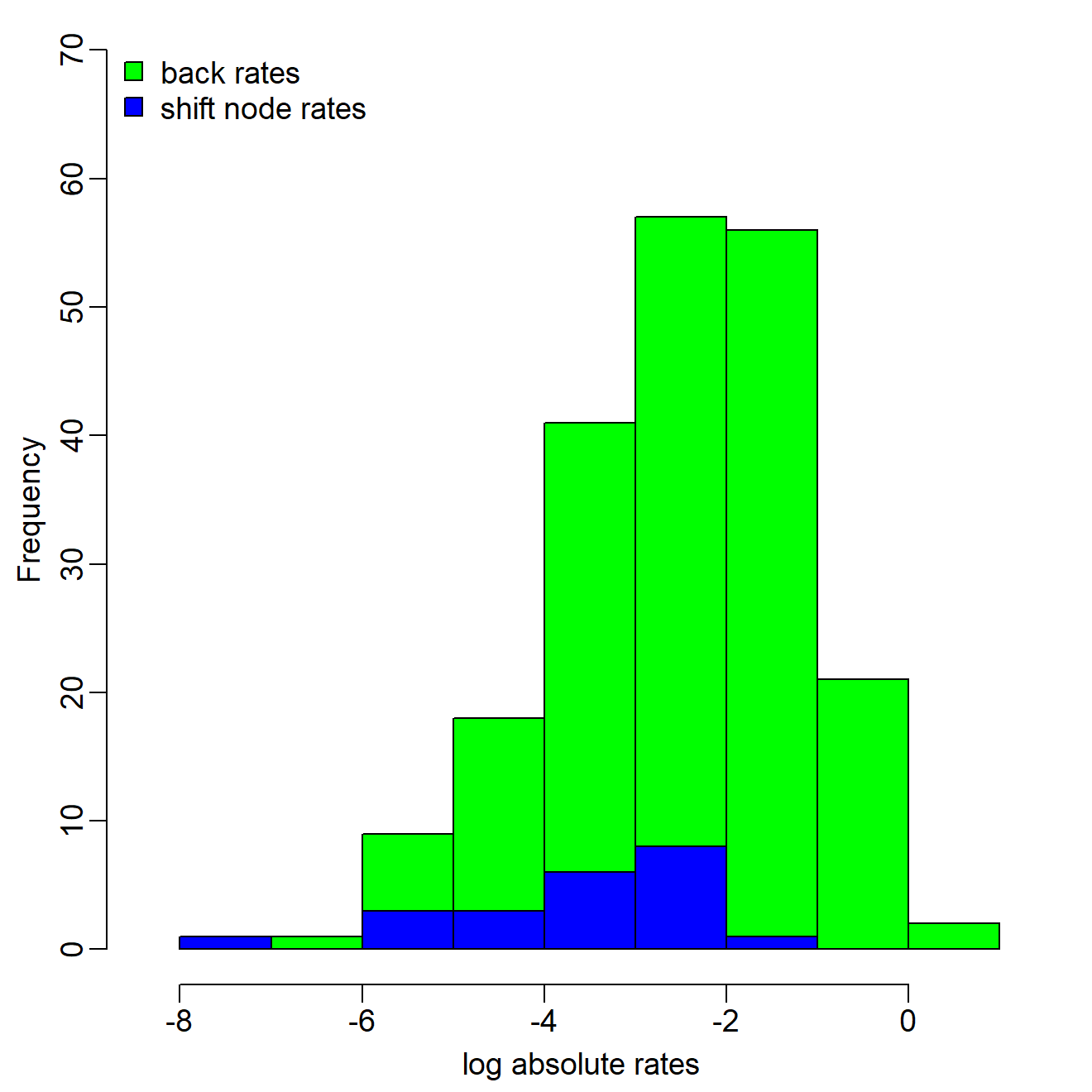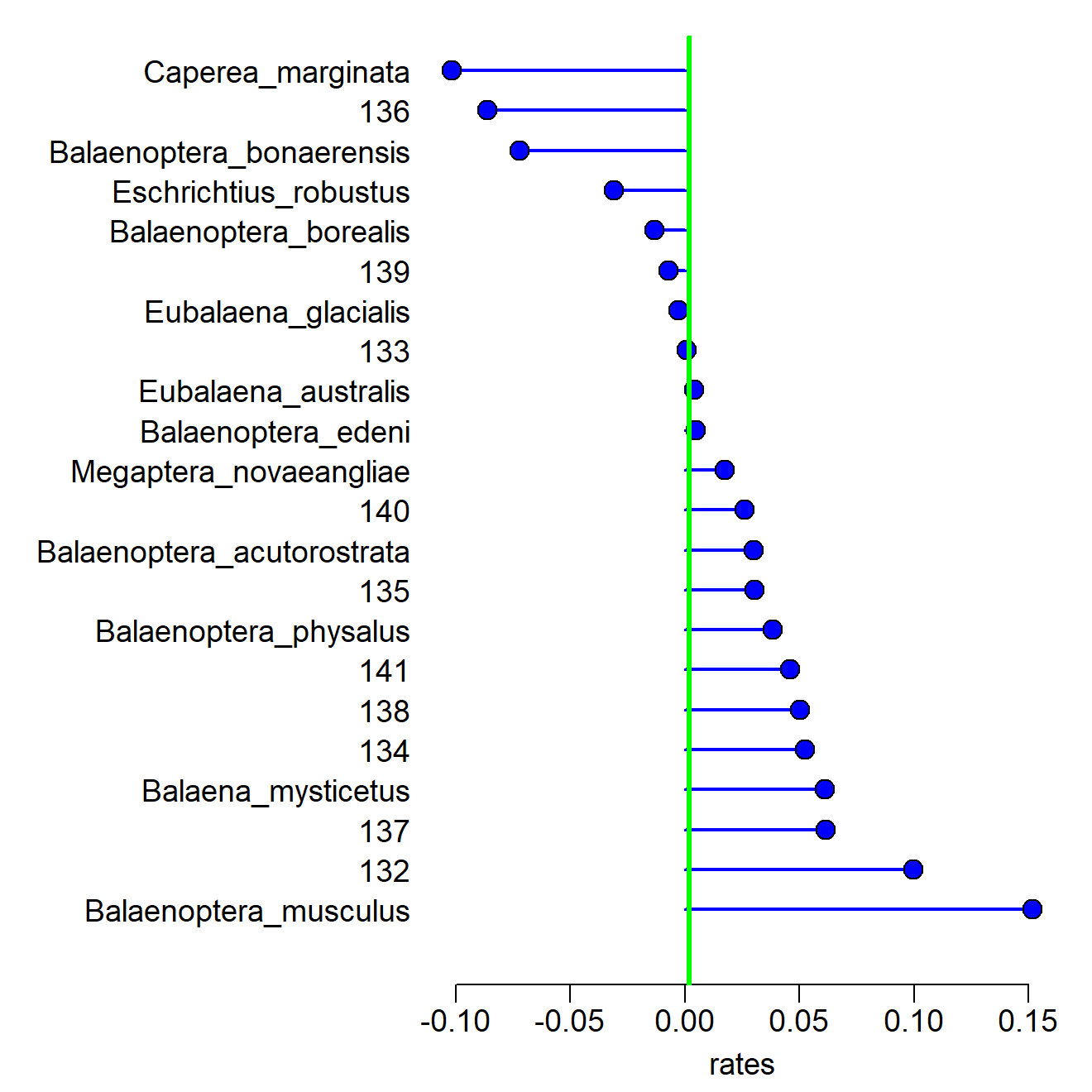# Customizing parameters
par(mar=c(3,3,1,1),mgp=c(1.7,0.5,0))
pRates$plotHist(hist.args=list(yaxt="n",ylab=NULL,col1="blue",col2="cyan"), legend.args = list(pch=21,pt.cex=2)) # Setting different pch and color for nodes and species colo<-rep("gray70",length(pLol)) colo[names(pLol)%in%treecet$tip.label]<-"cyan"
pchc<-rep(22,length(pLol))
pchc[names(pLol)%in%treecet$tip.label]<-21 par(mar=c(3,12,1,1),mgp=c(1.7,0.5,0)) pRates$plotLollipop(lollipop.args = list(pch=pchc,bg=colo,col=colo,cex=2,lwd=2),
line.args = list(col="deeppink2",lwd=4,lty=2))->pLol2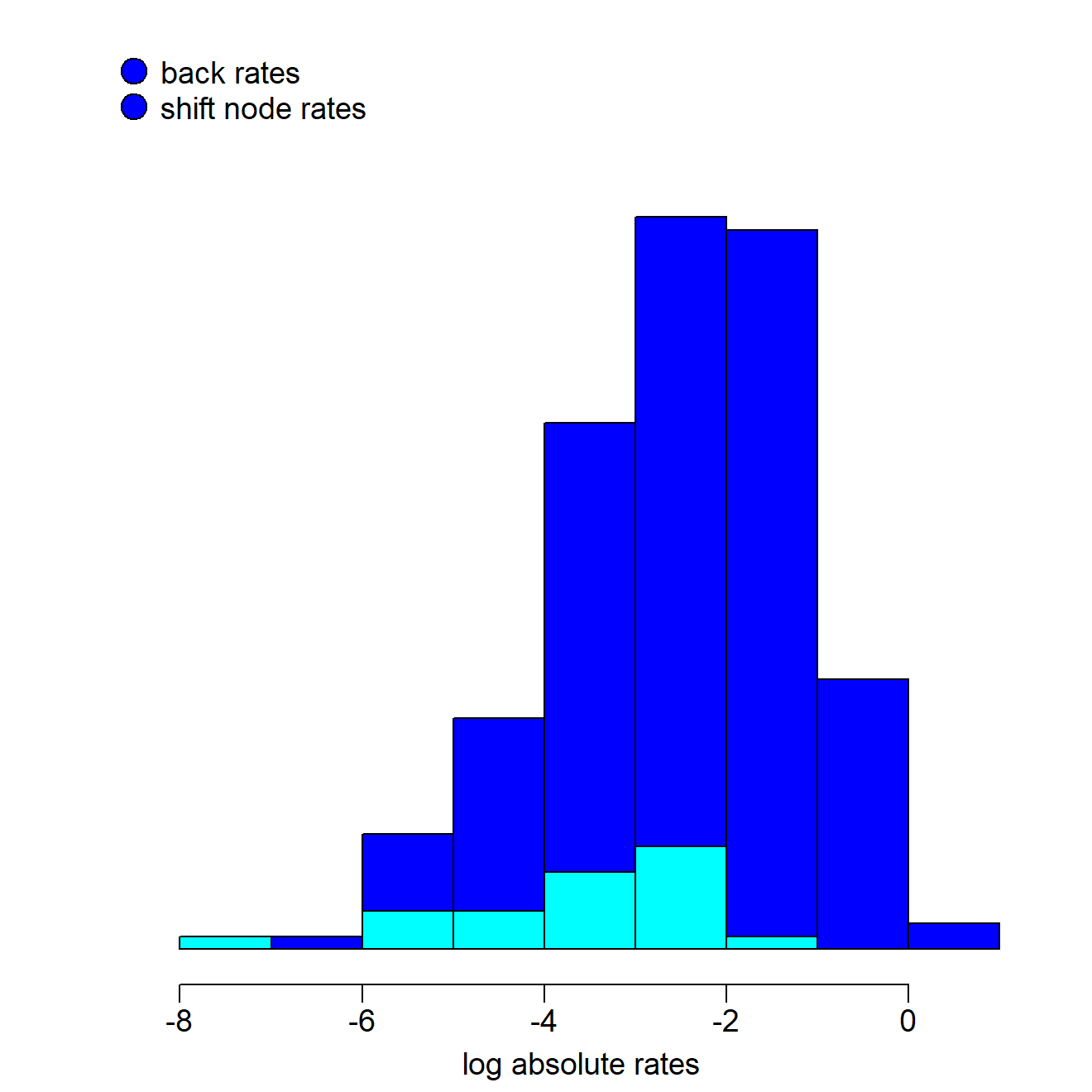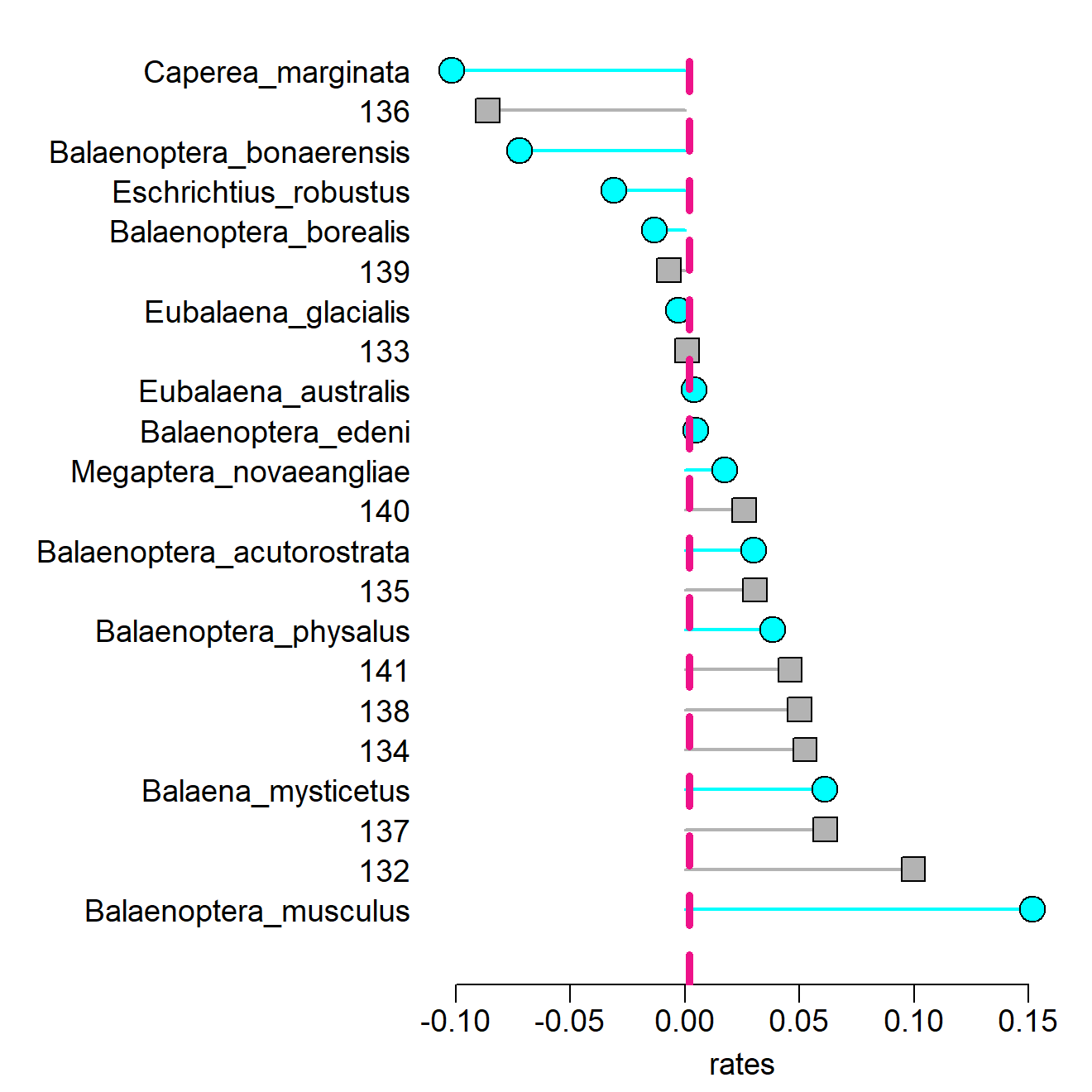## plotting search.trend results

Results for phenotypic and evolutionary rates trends through time returned by the function search.trend can be visualized by means of plotTrend. Such function takes as only object the output of search.trend and returns customized functions to produce plots of phenotypes (plotSTphen) and (rescaled) evolutionary rates (plotSTrates) versus time regressions on the entire tree. The plots generated by both these functions are basically made by a scatterplot representing the distribution of phenotypes/rates versus time, a polygon depicting the 95% CI of slopes generated according to the Brownian Motion (see search.trend for further details) and the regression line. Each of these elements can be customized by the user by specifying the arguments plot.args, polygon.args, and line.args. The only mandatory argument for plotSTphen and plotSTrates is the name or the number of the variable to plot.

### load the RRphylo example dataset including Felids tree and 4 mandible shape variables (PCs)
data("DataFelids")
DataFelids$treefel->treefel DataFelids$PCscoresfel->PCscoresfel

# Perform RRphylo and search.trend
cc<-2/parallel::detectCores()
RRphylo(treefel,PCscoresfel,clus=cc)->RRfel
search.trend(RRfel,PCscoresfel,clus=cc)->ST

# Visualize search.trend results
plotTrend(ST)->pTrend
# Using default options
par(mfrow=c(1,2),mar=c(4,3,3,1),mgp=c(1.5,0.5,0))
pTrend$plotSTphen("PC1") pTrend$plotSTrates(1) # This is for PC1 as well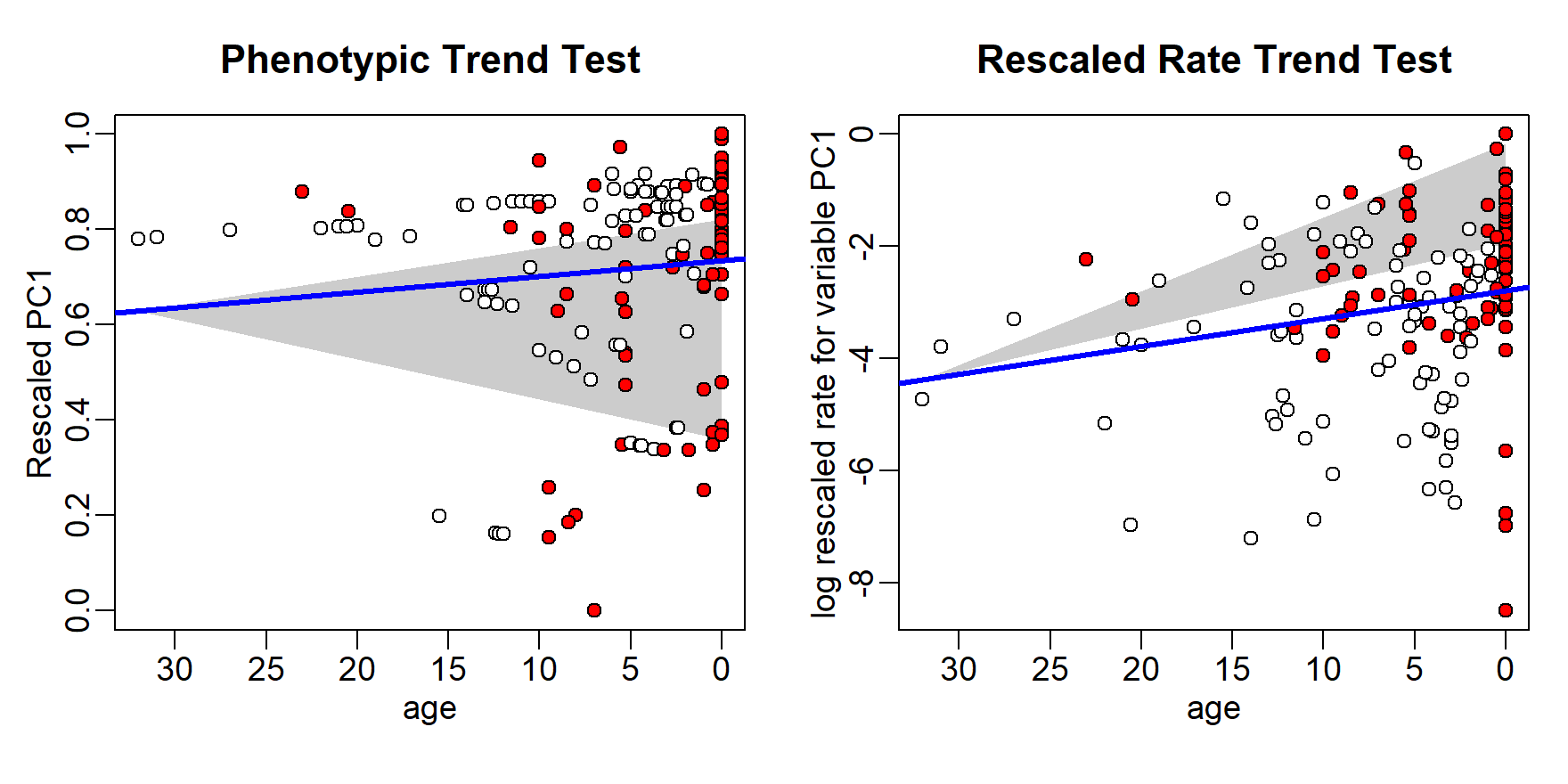## Customizing parameters
# Extracting nodes and tips descending from the most recent common ancestor of Felinae
library(phytools)
Felinae<-getDescendants(treefel,94)
Felinae[which(Felinae<=Ntip(treefel))]<-treefel$tip.label[Felinae[which(Felinae<=Ntip(treefel))]] # Setting different pch for Felinae only pchc<-rep(21,nrow(ST$trend.data$phenotypeVStime)) pchc[rownames(ST$trend.data$phenotypeVStime)%in%Felinae]<-6 par(mfrow=c(1,2),mar=c(4,3,3,1),mgp=c(1.5,0.5,0)) pTrend$plotSTphen("PC2",plot.args = list(pch=pchc,cex=1.3),
polygon.args = list(col="white",border="black",lwd=2,lty=3),
line.args = list(lty=4,col="purple3"))

# When multivariate data are used, a "rate" vector is calculated as the 2-norm
# vector of rates computed for each individual variable. The "total rate" can be plotted:
pTrend$plotSTrates("rate") # Equivalent to setting variable = 5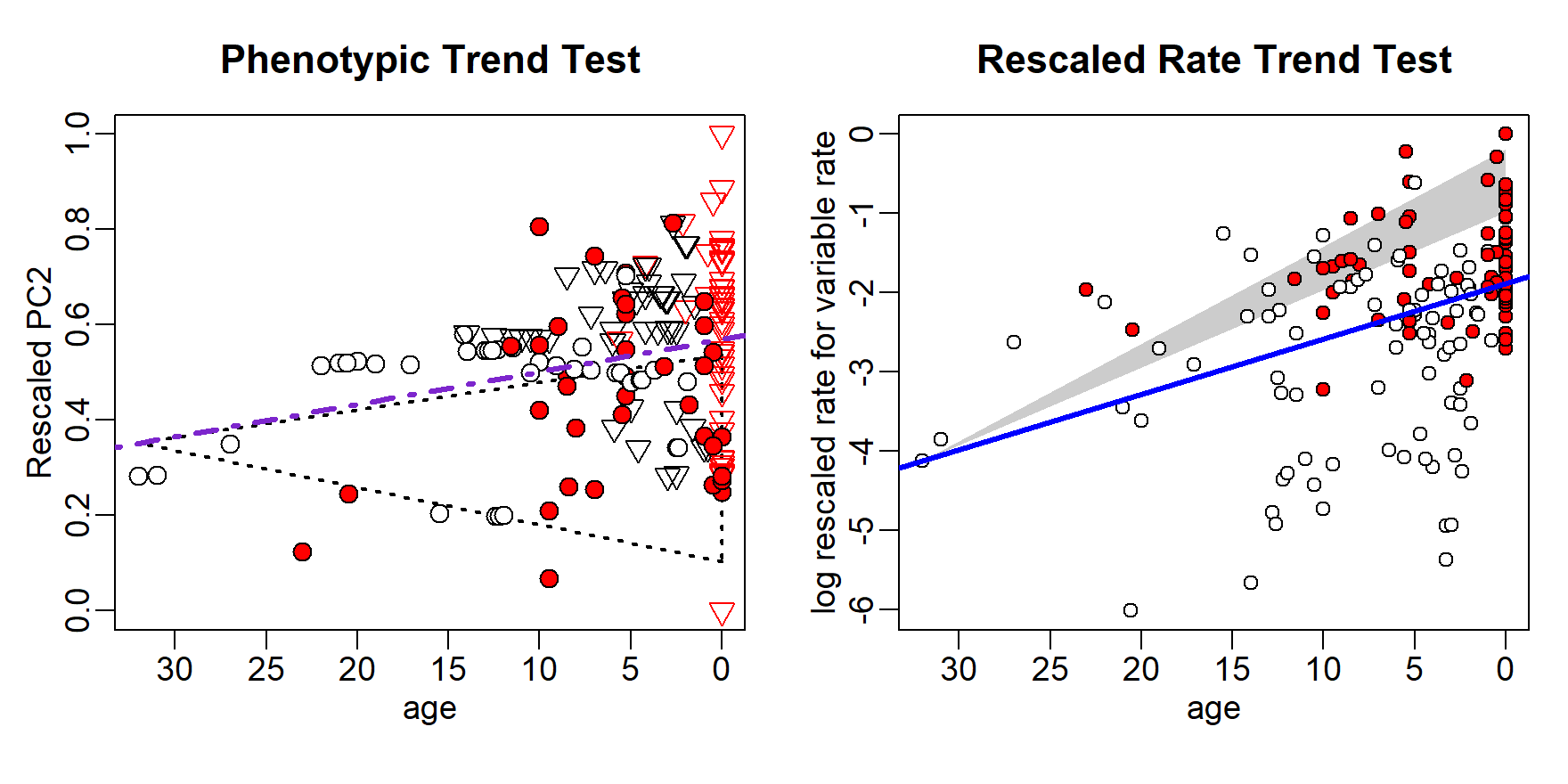If the output of search.trend also includes results for phenotypic and evolutionary rates trends trough time occurring at individual clades in the tree, plotTrend returns two additional functions to generate plots for individual nodes (plotSTphenNode and plotSTratesNode). As above, these functions allow the user to customize the plot by setting the parameters plot.args (for everything pertaining the scatterplot), lineTree.args and lineNode.args (for the regression lines for the entire tree and the clade, respectively), and node.palette (including as many colors as the number of nodes the plot should be produced for). Mandatory arguments are the variable and the indices or numbers of nodes to plot (up to 9 nodes at the same time). An argument peculiar to these functions (listed in plot.args) is pch.node, which can be used to set different pch for each node. # Perform search.trend setting Smilodontini and Pantherini as individual clades search.trend(RRfel,PCscoresfel,node=c(129,154),clus=cc)->STclades # Visualize search.trend results plotTrend(STclades)->pTrend2 # Using default options par(mar=c(4,3,3,1),mgp=c(1.5,0.5,0)) pTrend2$plotSTphenNode("PC1",node=1:2)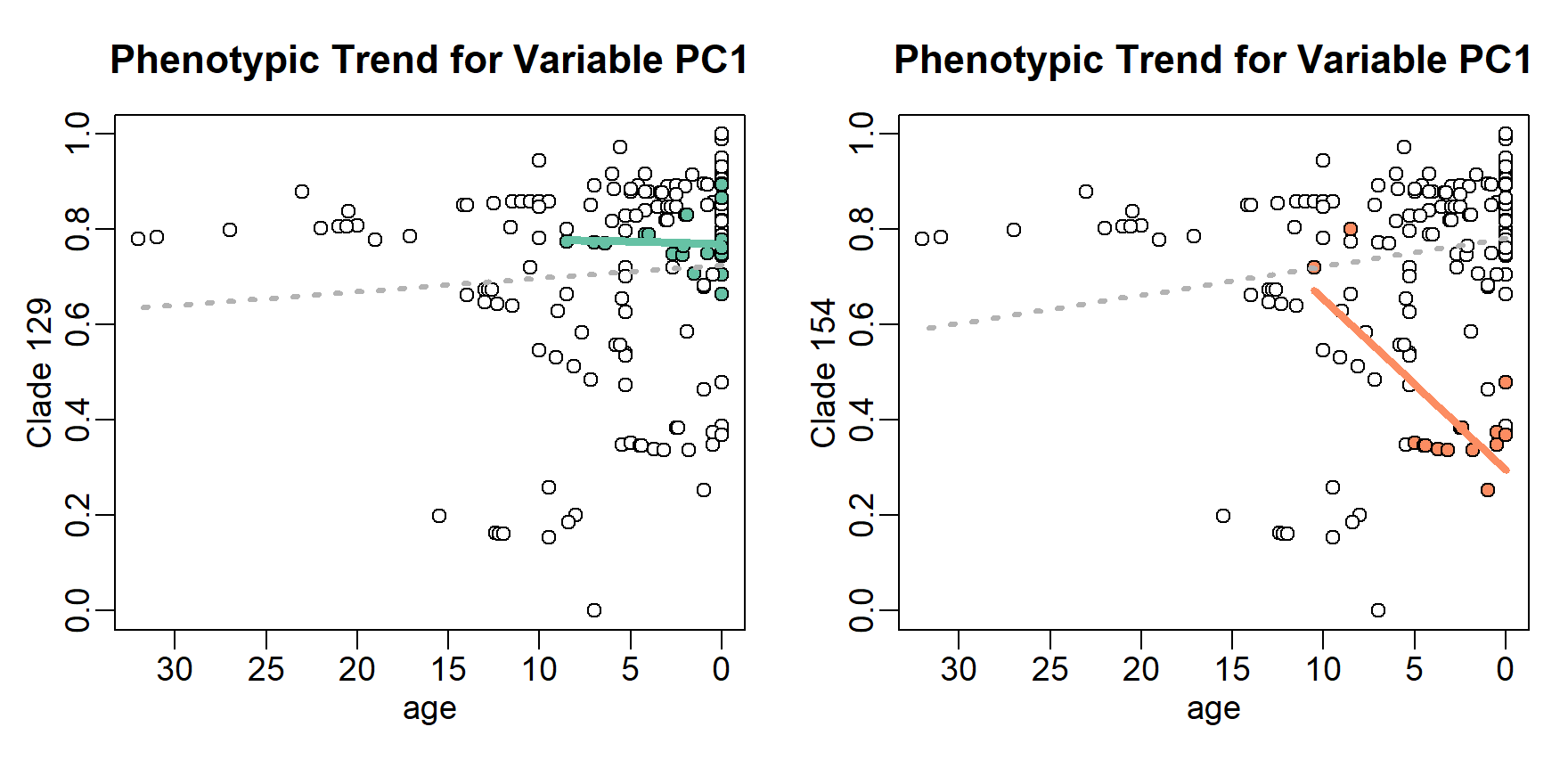pTrend2$plotSTratesNode("PC1",node=c(154,129)) # This is the same as indicating node= 2:1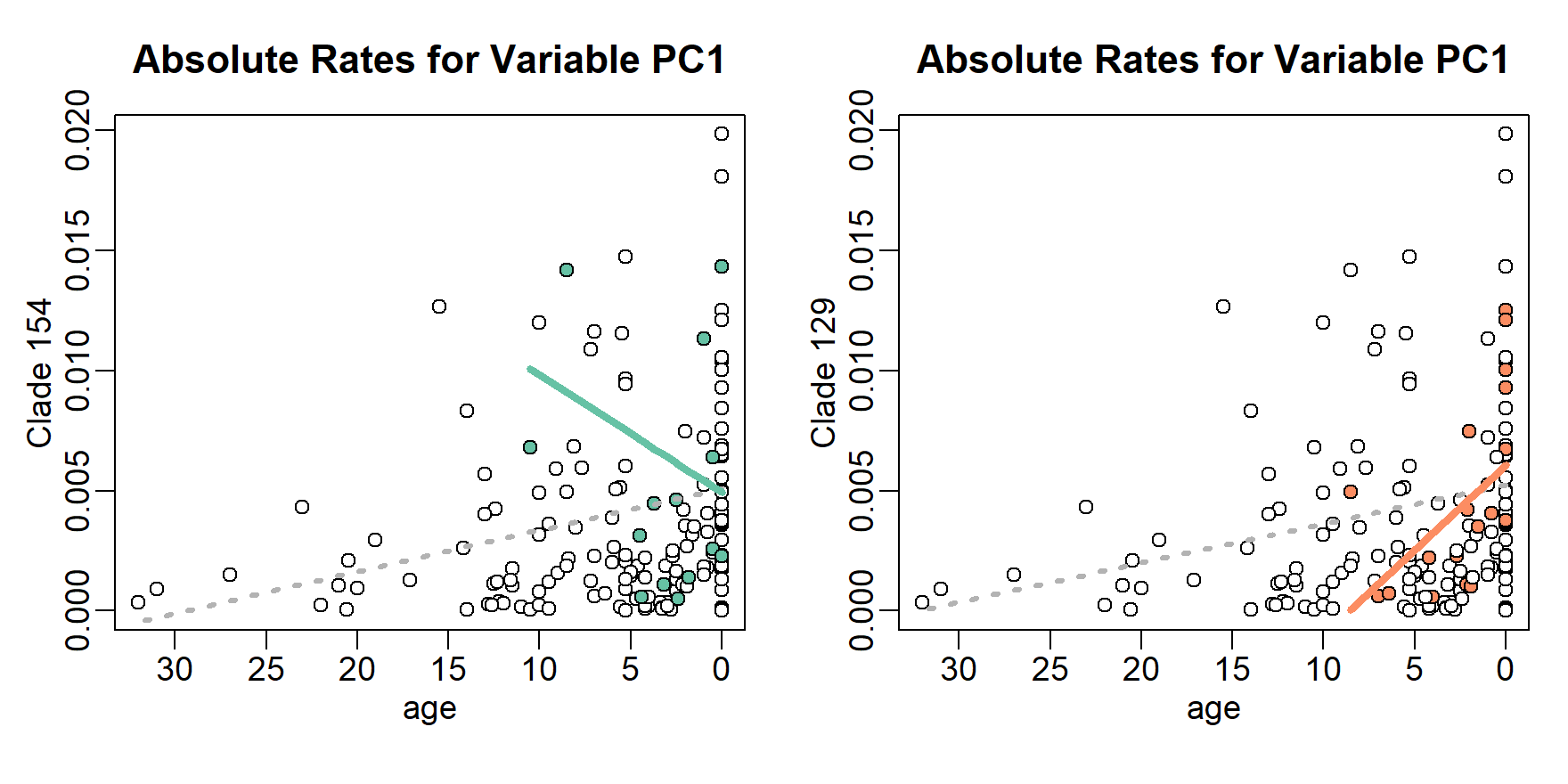## Customizing parameters par(mar=c(4,3,3,1),mgp=c(1.5,0.5,0)) pTrend2$plotSTphenNode("PC2",node=1:2,
plot.args = list(pch.node=c(23,24),pch=1,col="gray70",cex=1.2),
lineTree.args = list(col="black",lwd=3),lineNode.args = list(lwd=5),
node.palette = c("orangered","chartreuse"))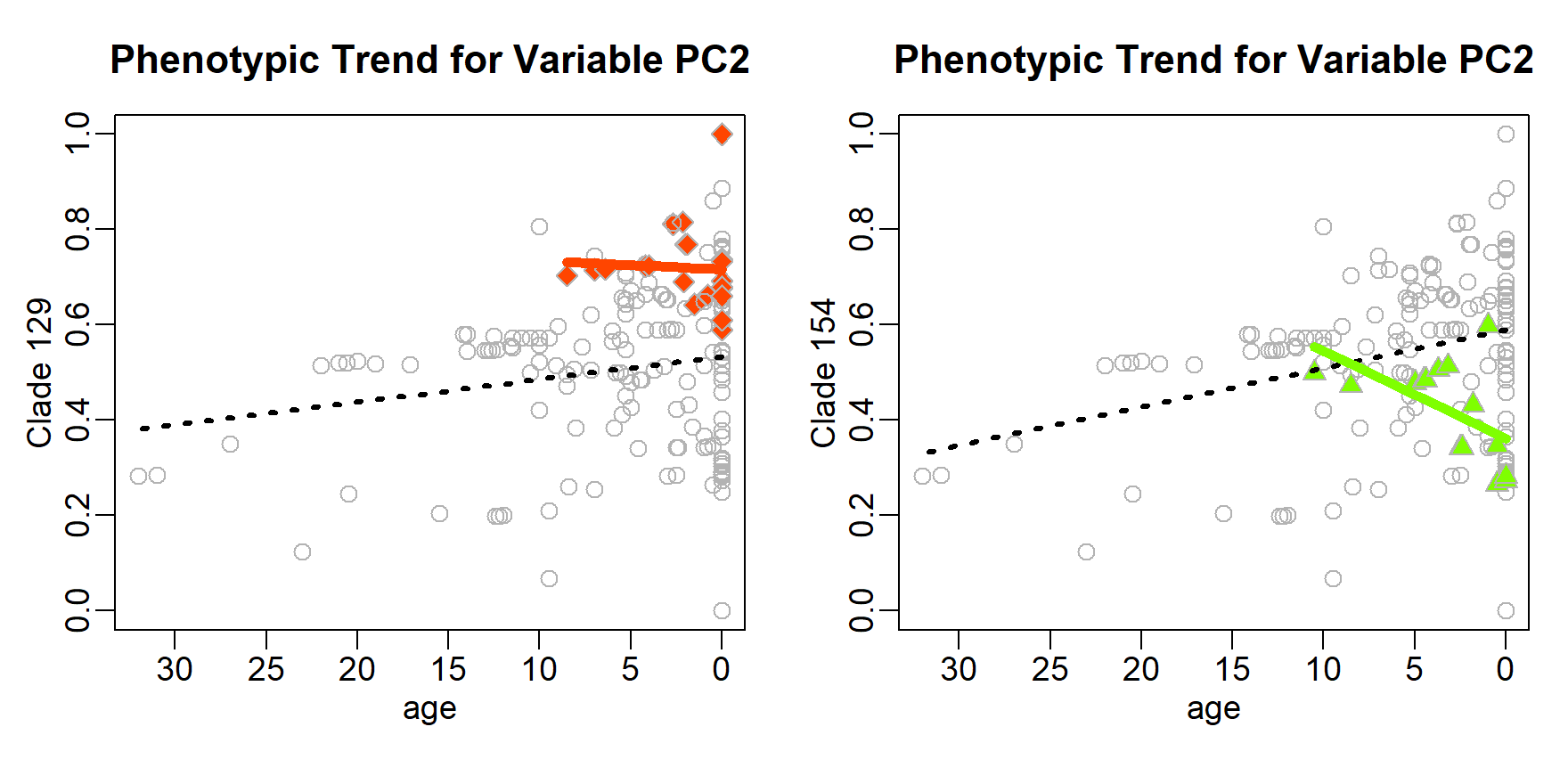pTrend2$plotSTratesNode("rate",node=c(154,129), plot.args = list(pch.node=c(5,6),pch=16,col="gray70",cex=1.2,lwd=2), lineTree.args = list(col="gold",lwd=3,lty=4), lineNode.args = list(lwd=5), node.palette = c("deeppink","cyan2"))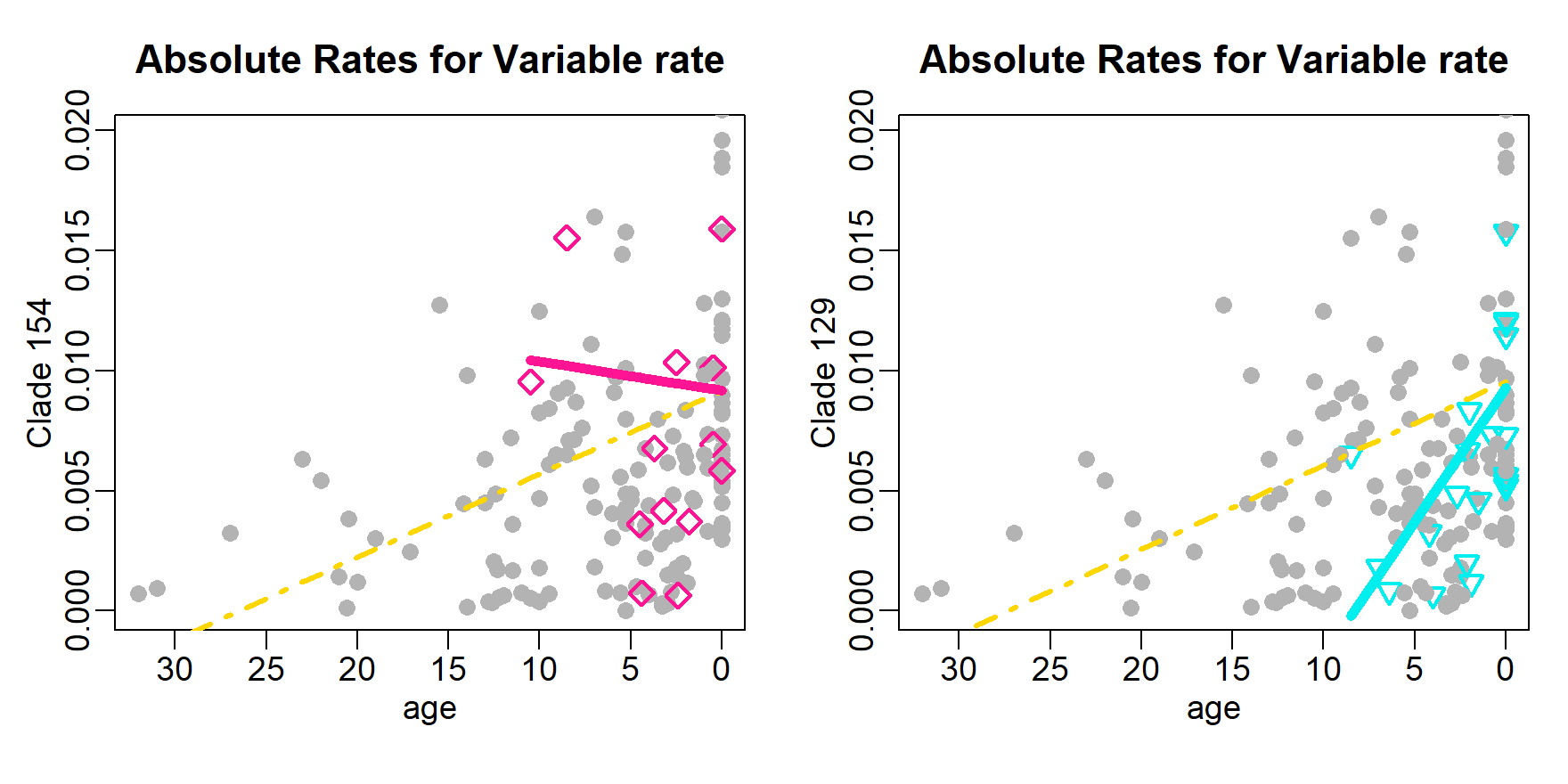## plotting search.shift results Evolutionary rate shifts located on the tree by the function search.shift can be visualized by means of plotShift. Such function takes as mandatory objects the outputs of RRphylo and search.shift and returns customized functions to produce plots of evolutionary rates shifts occurring at clade level (plotClades) or sparse across the tree (plotStates), depending on the status.type set when performing search.shift. In the latter case (i.e. status.type = "sparse") a further state argument must be provided to plotShift. plotClades highlights the shifting clades onto the phylogenetic tree by drawing colored circles on their Most Recent Common Ancestors (MRCA). The radii of such circles are directly proportional to the absolute value of absolute rate difference (see search.shift for further details), so that a circle for a clade whose mean absolute rates “shifts” more from the mean absolute rates of the tree is larger than a circle for a clade with a smaller difference. Plots for the tree and the circles can be customized by the user by specifying the arguments tree.args and symbols.args, respectively. For example, the user can choose different color options than the standard blue/red for positive/negative shifts, by setting symbols.args=list(fg=c(pos="color for positive shift",neg="color for negative shift")), or provide a vector of as many colors as the number of shifting clades. The same applies to the symbols argument “bg”. ### load the RRphylo example dataset including Ornithodirans tree, body mass and locomotory type data("DataOrnithodirans") DataOrnithodirans$treedino->treedino
DataOrnithodirans$massdino->massdino DataOrnithodirans$statedino->statedino

# Perform RRphylo and search.shift under status.type="clade"
RRphylo(tree=treedino,y=massdino)->dinoRates

# Visualize search.shift results
plotShift(RR=dinoRates,SS=SSauto)->plotSS
# Using default options
plotSS$plotClades()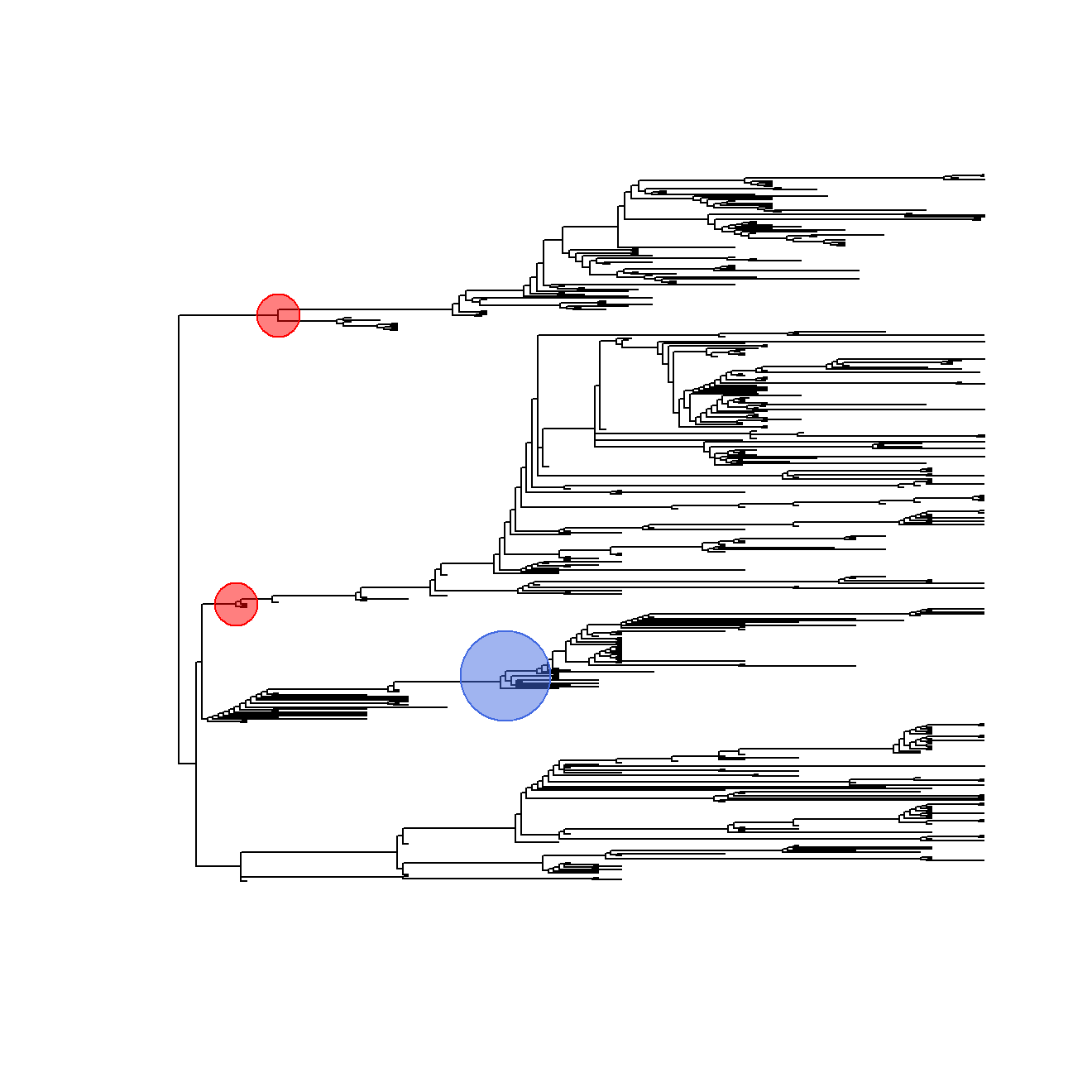## Customizing parameters # Setting different colors for positive and negative shift plotSS$plotClades(tree.args=list(no.margin=TRUE,type="fan"),
symbols.args=list(lwd=2,fg=c(pos="gold",neg="green"),
bg=scales::alpha("grey70",0.3)))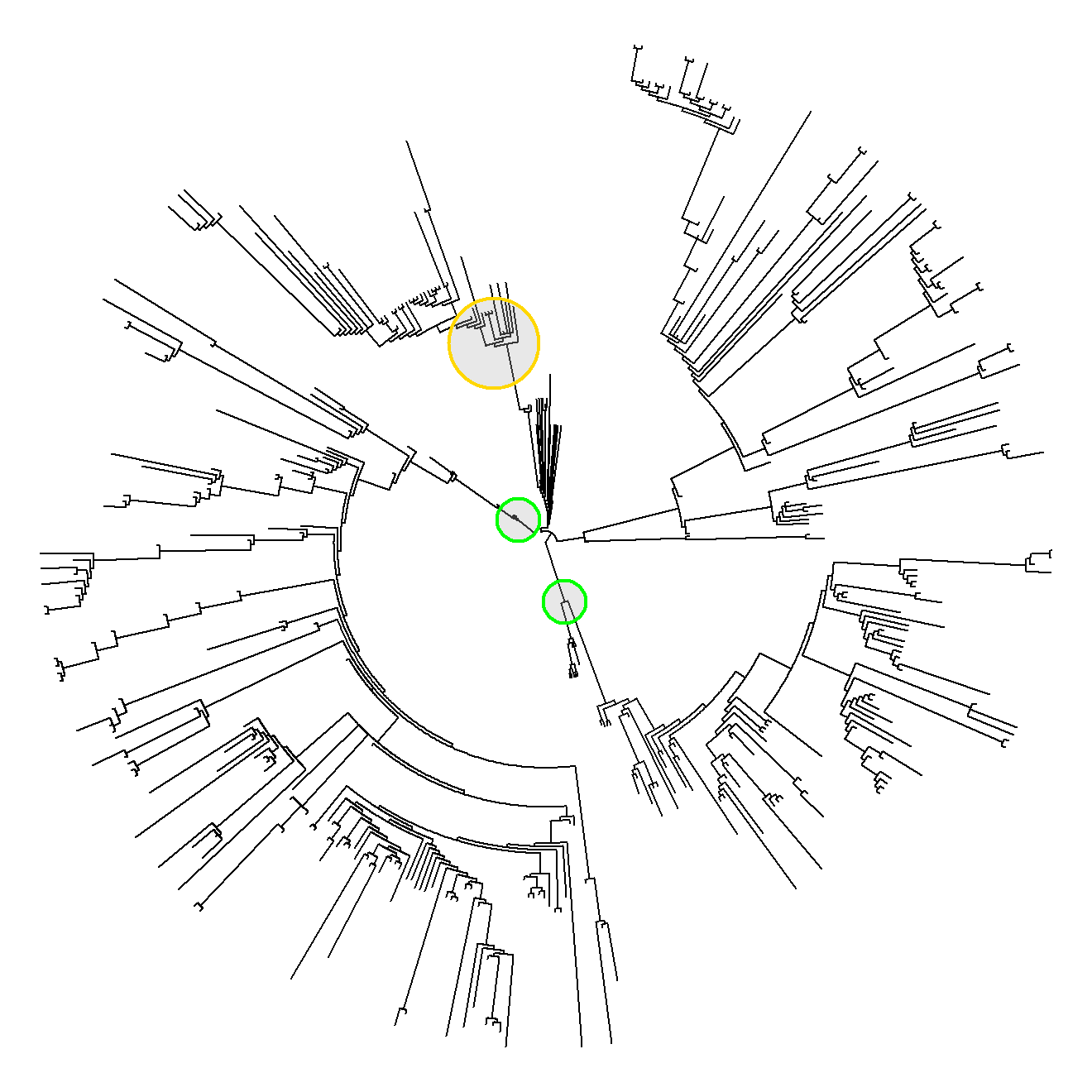# Setting different colors for each shifting clade
plotSS$plotClades(tree.args=list(no.margin=TRUE), symbols.args=list(lwd=2,fg=NA,bg=scales::alpha(c("red","green","blue"),0.3)))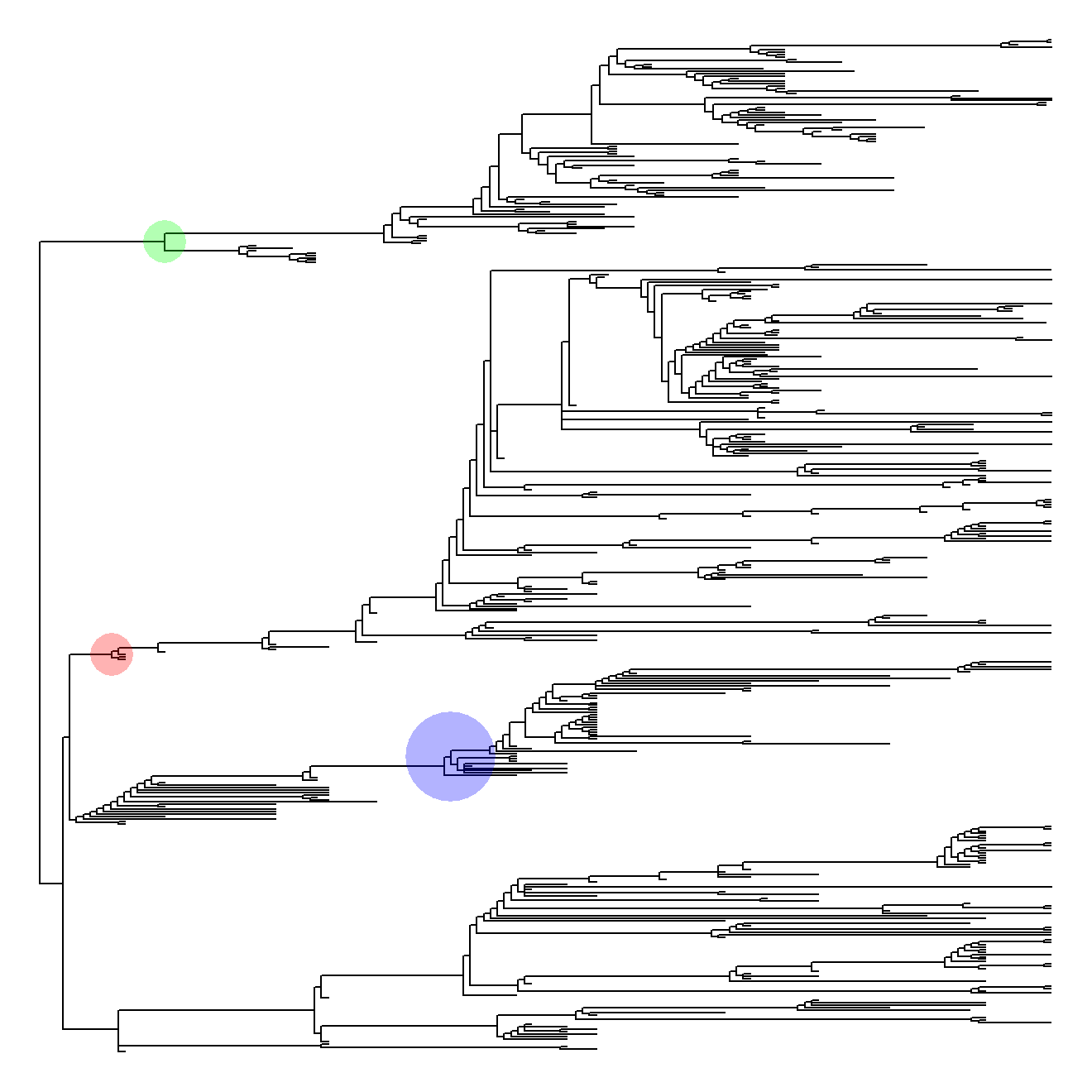If search.shift was performed under status.type = "sparse", the function plotStates (returned by plotShift) plots the states onto the phylogenetic tree and prints into the legend the direction (i.e. positive or negative) of significant shifts for each state. Plots for the tree, the points, and the legend can be customized by the user by specifying the arguments tree.args, points.args, and legend.args respectively. # Perform RRphylo and search.shift under status.type="sparse" search.shift(RR=dinoRates,status.type= "sparse",state=statedino)->SSstate # Visualize search.shift results plotShift(RR=dinoRates,SS=SSstate,state=statedino)->plotSS2 # Using default options plotSS2$plotStates()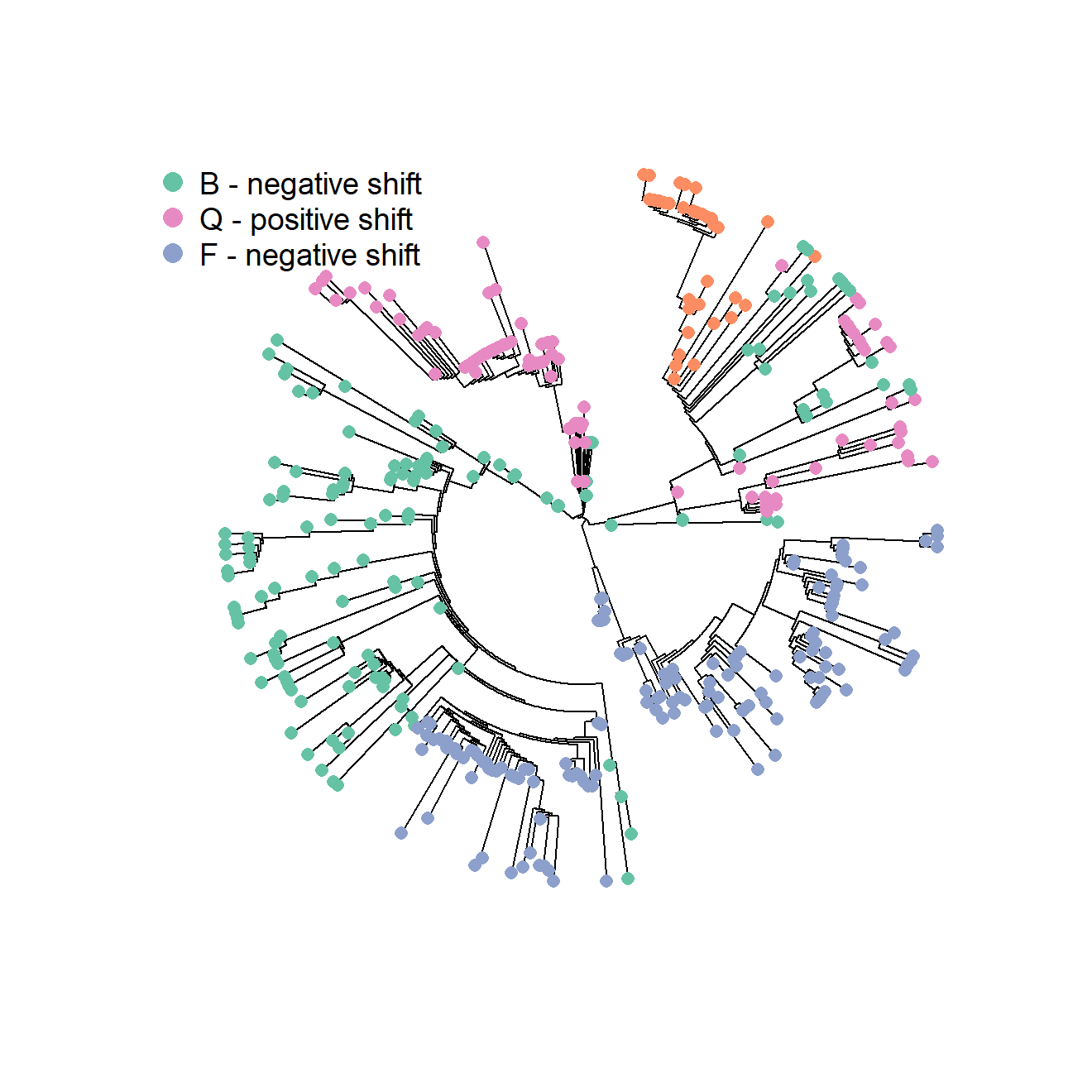## Customizing parameters
# Setting customized colors and pch for different states and suppressing the legend
plotSS2$plotStates(tree.args=list(no.margin=TRUE,type="phylogram"), points.args=list(bg=c("gold","forestgreen","royalblue","white"), col=c("black","black","black","orangered"), pch=c(21,22,24,11)),legend.args=NULL) # Setting customized colors and pch for different states and suppressing the legend plotSS2$plotStates(tree.args=list(no.margin=TRUE,type="phylogram"),
points.args=list(bg=c("gold","forestgreen","royalblue","white"),
col=c("black","black","black","orangered"),
pch=c(21,22,24,11)),legend.args=list(pch=21))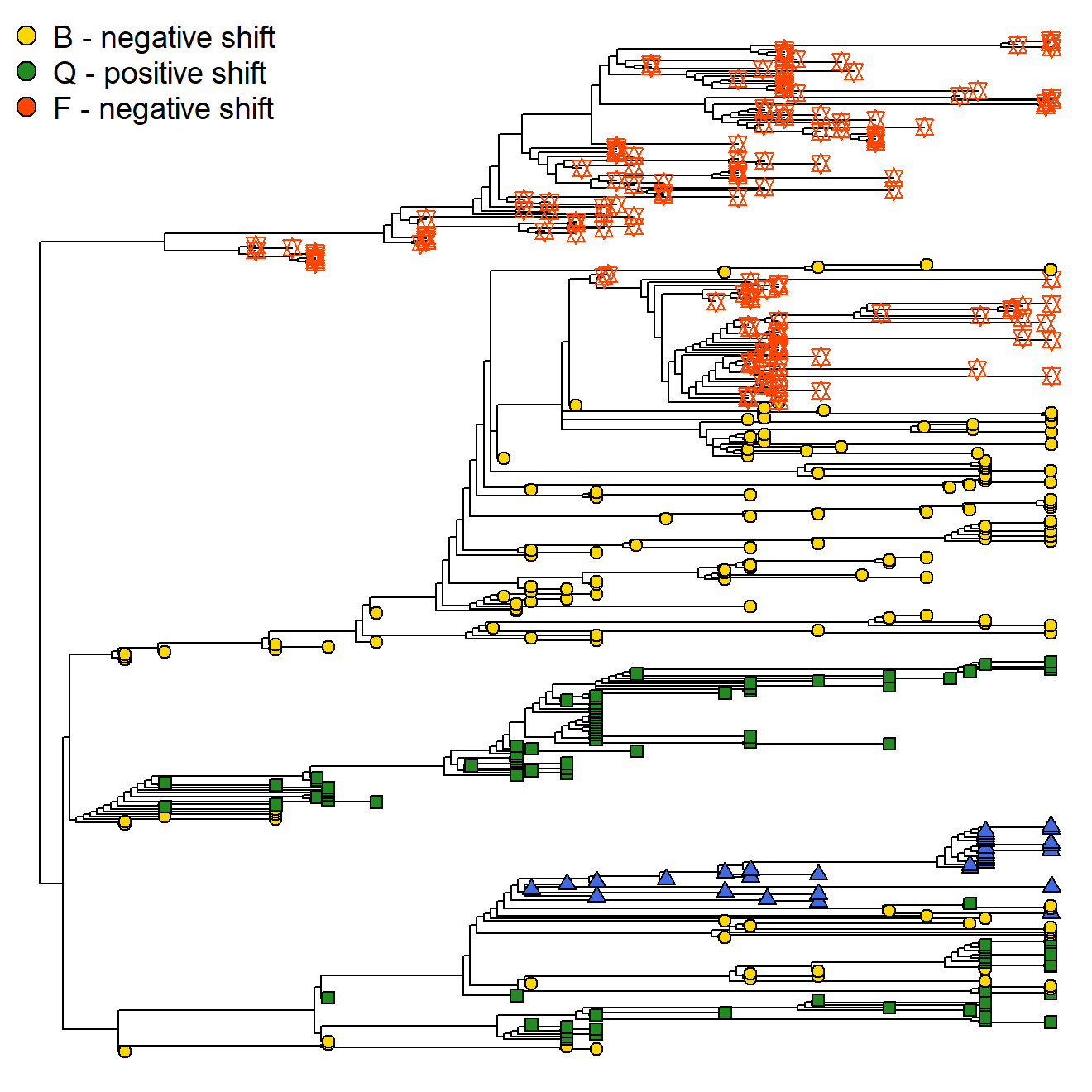## plotting search.conv results

Instances of phenotypic convergence detected on the tree by the function search.conv can be visualized by means of plotConv. Such function takes as mandatory objects the outputs of search.conv, the multivariate phenotype (y) used to perform search.conv, and the index of result to plot (variable), and returns customized functions to produce plots of convergence occurring at clade level or related to some discrete category scattered within the tree, depending on the way search.conv was performed. In the latter case (convergence within/between states) a further state argument must be provided to plotConv.

When convergence between clades is inspected, four functions are returned. plotHistTips and plotHistAces generate histograms of the euclidean distances between phenotypic vectors of all possible pairs of tips/nodes within the phylogeny, and draw a vertical line representing the real distance between tips/nodes within convergent clade pair (it is the mean distance in the case of tips). Histogram and line characteristics can be customized by the user by specifying the arguments hist.args and line.args. The function plotPChull generates a PC1/PC2 plot (obtained by performing a PCA of the species phenotypes) with convergent clades represented by colored convex hulls, and the mean phenotype and the ancestral characters for such clades indicated by customizable symbols. The arguments passed to plotPChull allow to modify scatterplot elements (plot.args), convex hull charateristics (chull.args), and the points for mean phenotypes (means.args) and ancestral characters (ace.args). The function plotTraitgram produces a modified traitgram plot (see package picante) where converging clades are highlighted by different colors. The colors for branches belonging to converging clades and for other branches can be customized by indicating the arguments colNodes and colTree, respectively.

### load the RRphylo example dataset including Felids tree, 4 mandible shape variables (PCs),
### and a category indicating whether or not the species shows sabertooth morphology
data("DataFelids")
DataFelids$PCscoresfel->PCscoresfel DataFelids$treefel->treefel
DataFelids$statefel->statefel # Perform RRphylo and search.conv between a pair of clades cc<-2/parallel::detectCores() RRphylo(treefel,PCscoresfel,clus=cc)->RRfel search.conv(RR=RRfel, y=PCscoresfel, nodes=c(85,155),clus=cc)->sc_clade ## Visualize search.conv results # Set variable = 1 to see results for the pair "85/155" (the first element in # sc_clade$average distance from group centroids)
plotConv(SC=sc_clade, y=PCscoresfel, variable=1, RR = RRfel)->plotSC
# Using default options
par(mfrow=c(1,2))
plotSC$plotHistTips() plotSC$plotHistAces()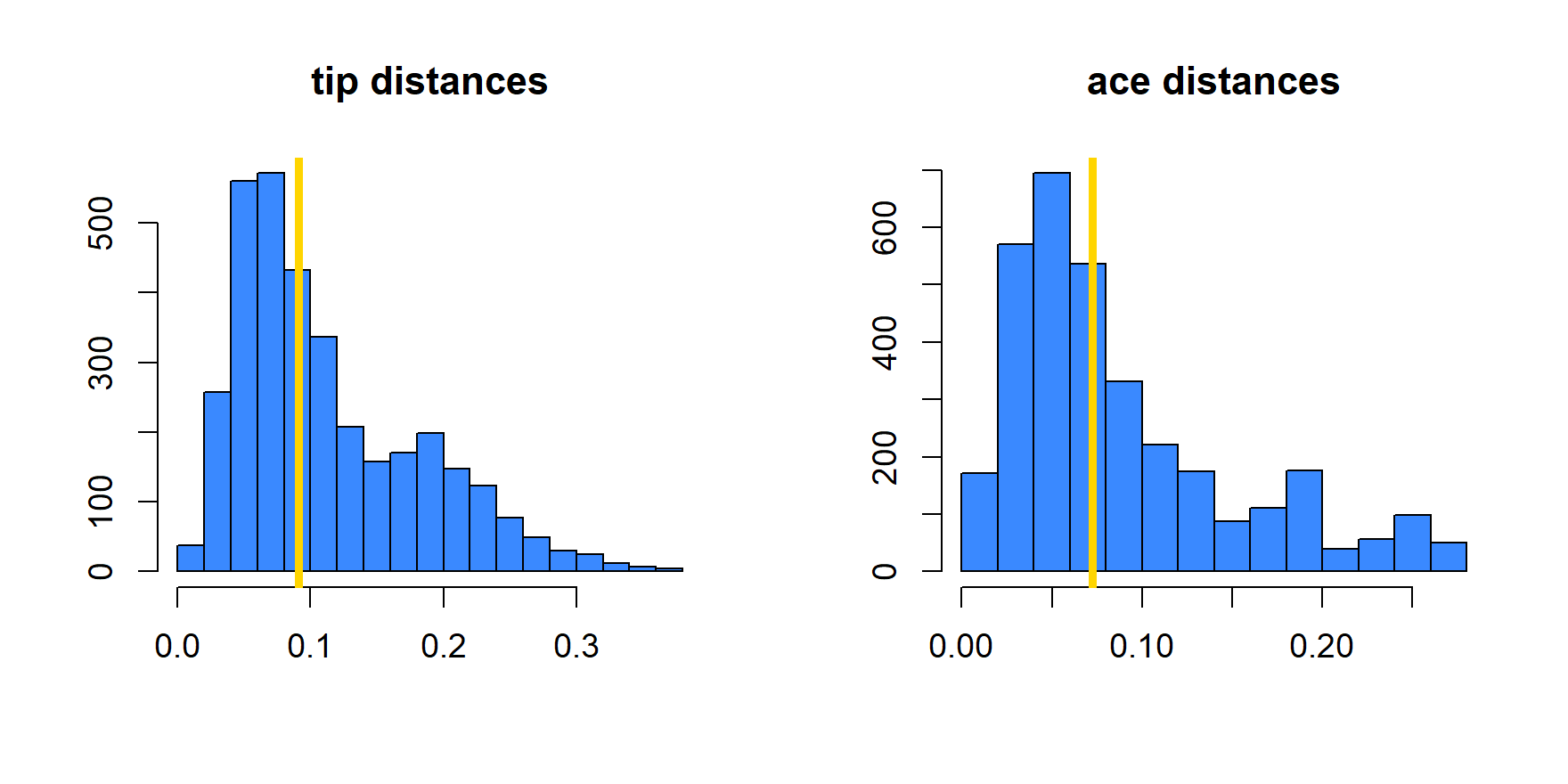plotSC$plotPChull() plotSC$plotTraitgram()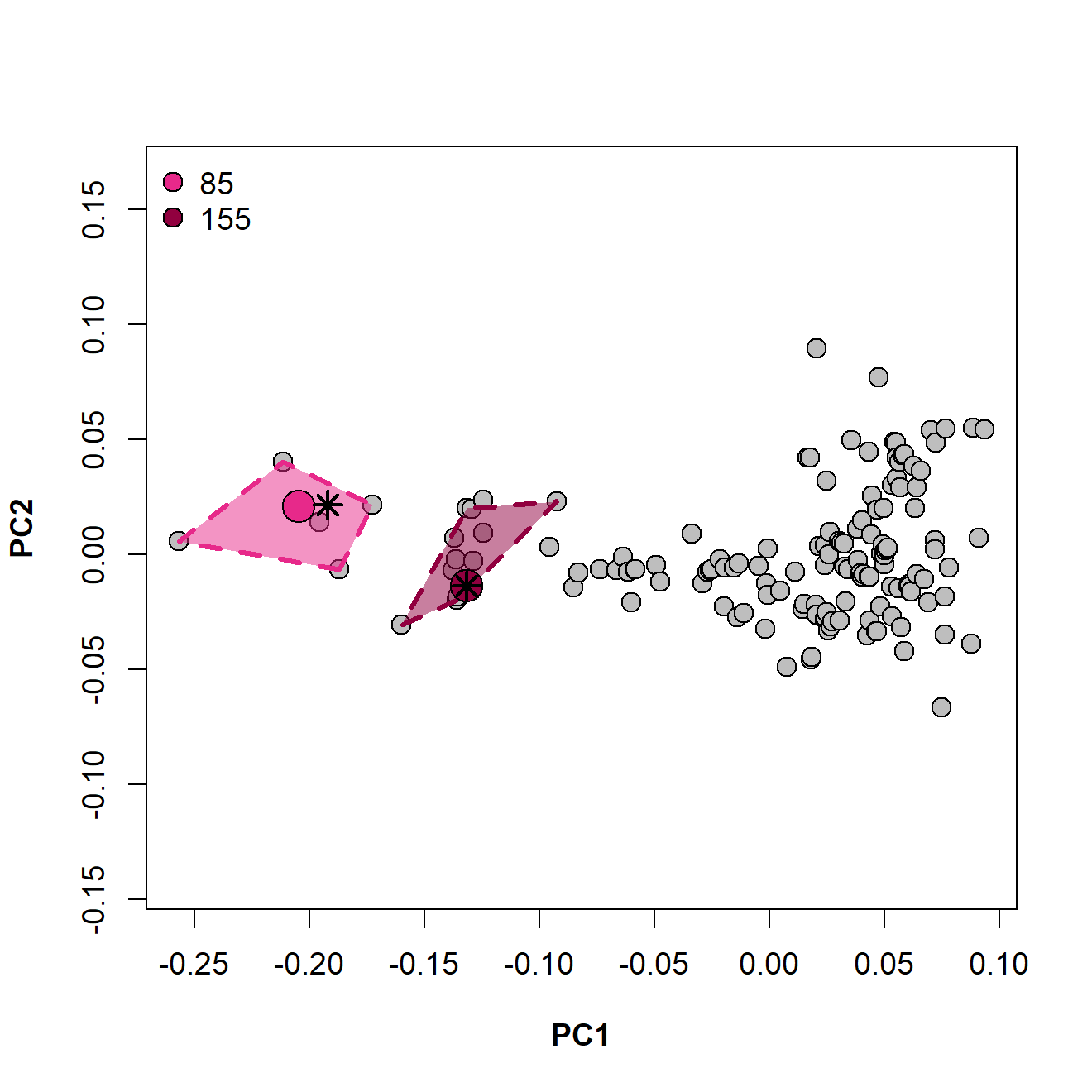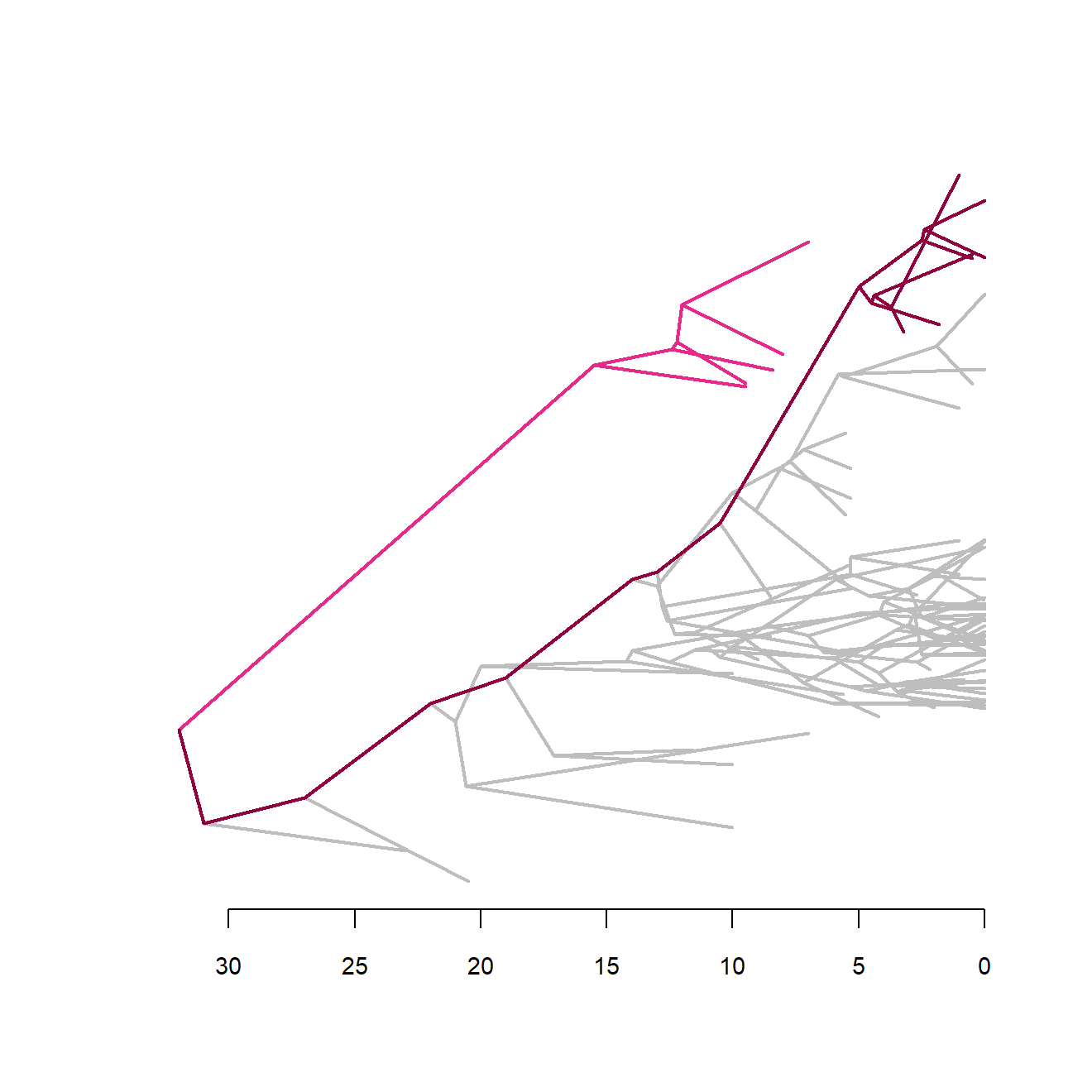## Customizing parameters
# Set variable = 2 to see results for the pair "86/156" (the second element in
# sc_clade$average distance from group centroids) plotConv(SC=sc_clade, y=PCscoresfel, variable=2, RR = RRfel)->plotSC par(mfrow=c(1,2)) plotSC$plotHistTips(hist.args = list(col="gray80",yaxt="n",cex.axis=0.8,cex.main=1.5),
line.args = list(lwd=3,lty=4,col="purple"))
plotSC$plotHistAces(hist.args = list(col="gray80",cex.axis=0.8,cex.main=1.5), line.args = list(lwd=3,lty=4,col="gold"))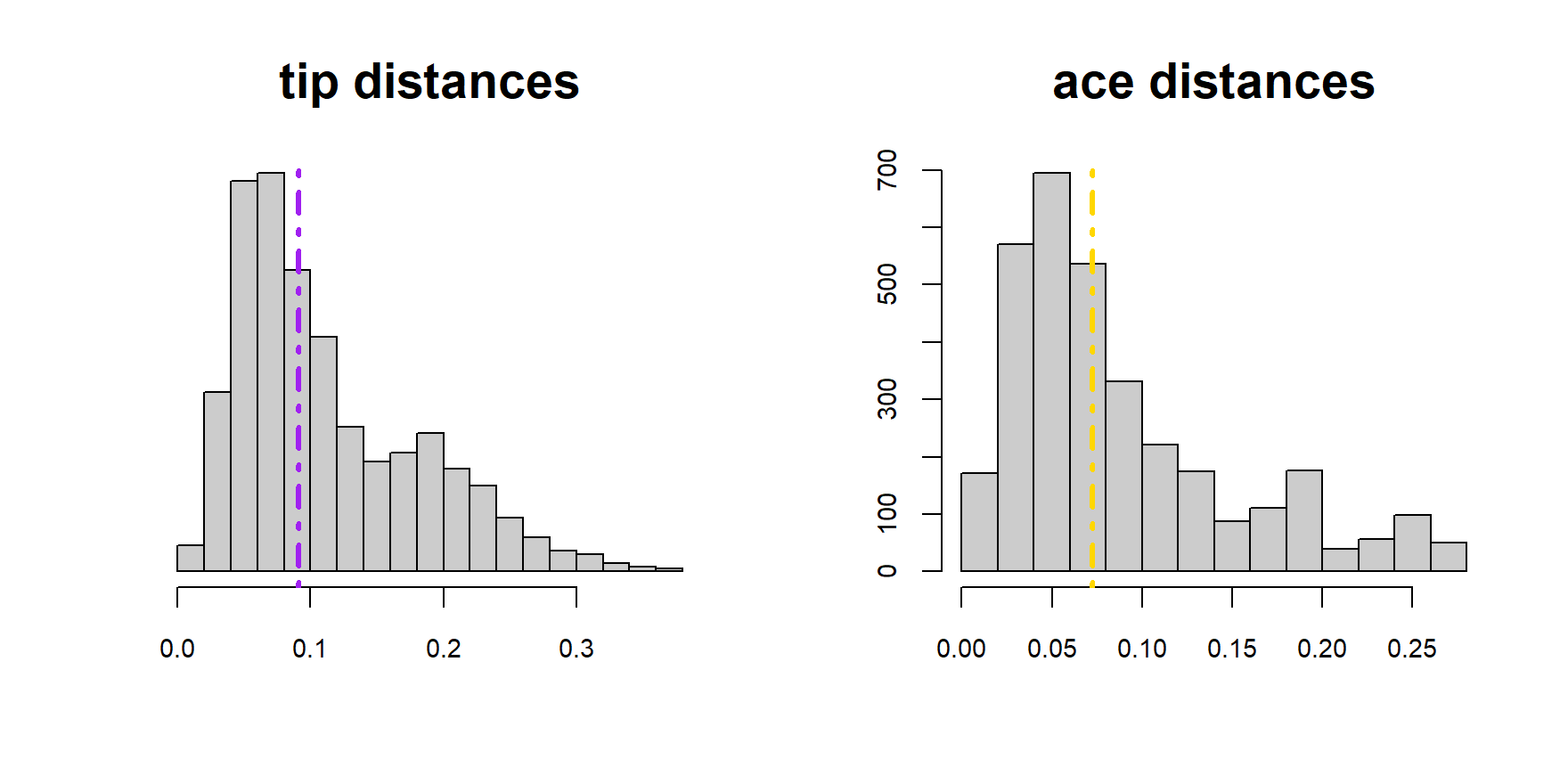plotSC$plotPChull(chull.args = list(border=c("cyan","magenta"),lty=1),
means.args = list(pch=c(21,22),cex=3,bg=c("cyan2","magenta2")),
ace.args=list(pch=c(7,10)),legend.args = list(pch=c(24,11),bty="o",x="top"))
plotSC$plotTraitgram(colTree = "gray70",colNodes = c("cyan","magenta"),yaxt="s")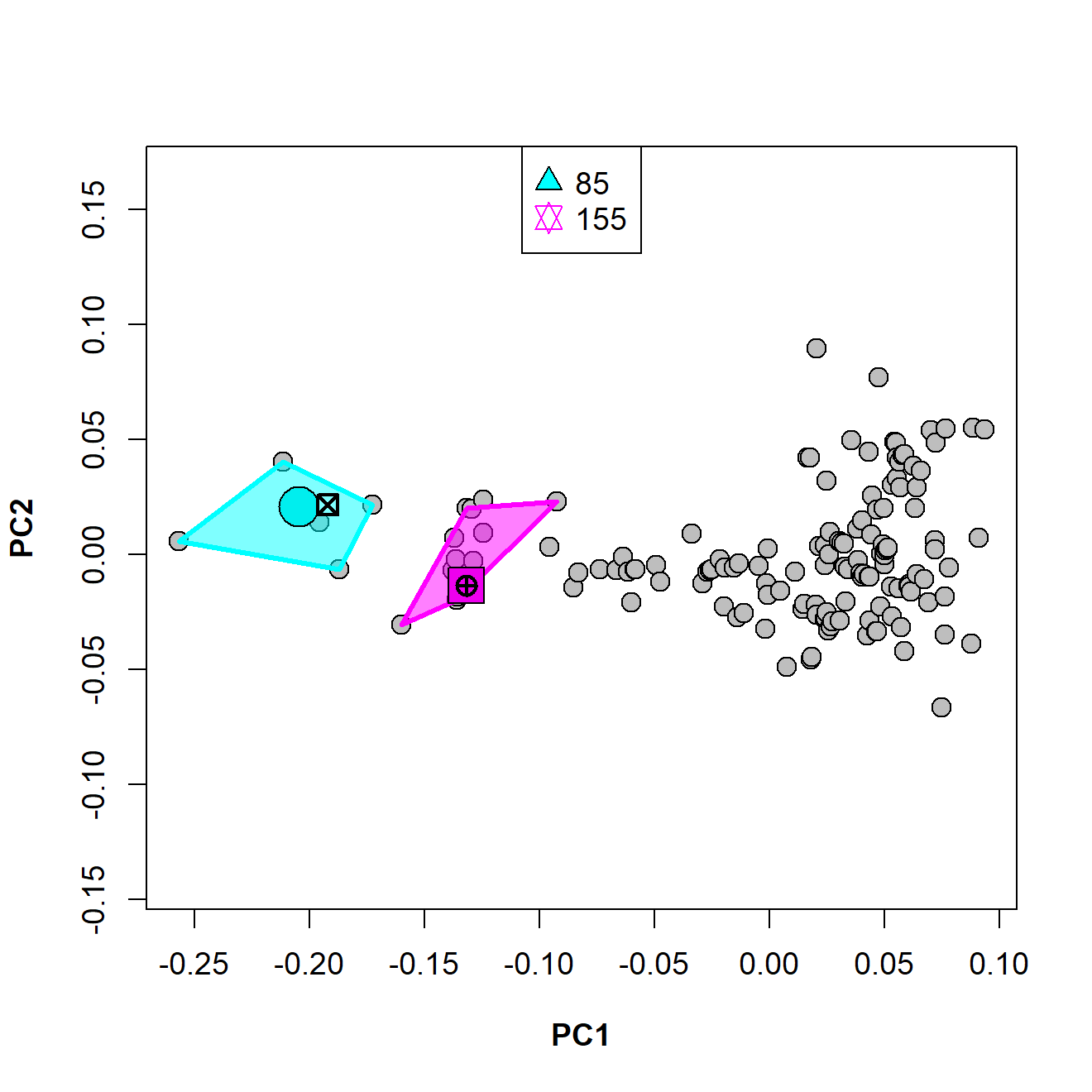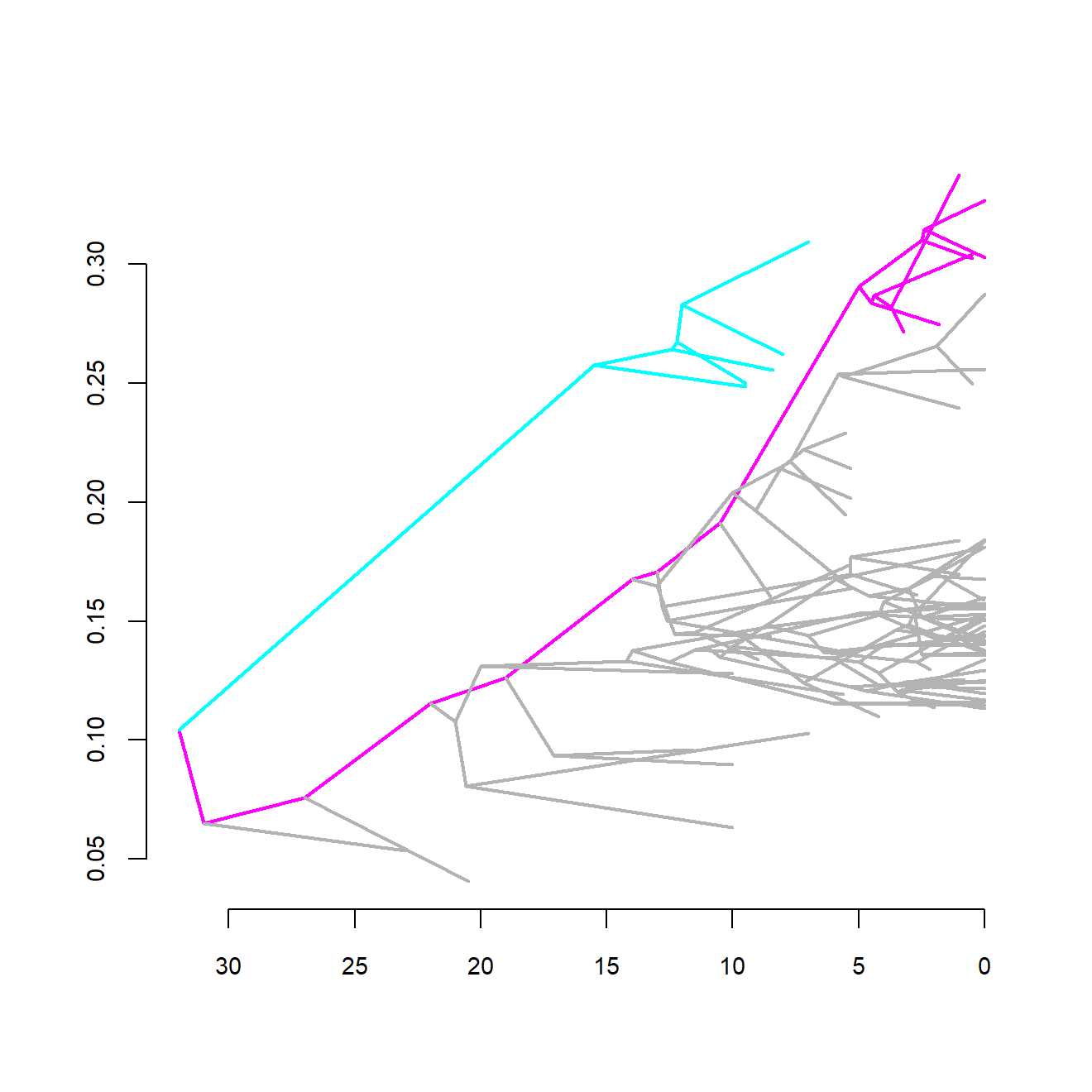When convergence between states is tested, plotConv returns two functions. Similarly to the ‘clade case’, plotPChull generates a PC1/PC2 plot (obtained by performing a PCA of the species phenotypes) with different states enclosed by colored convex hulls. The plot is entirely customizable by setting the arguments plot.args,chull.args, points.args, and legend.args. If the state vector includes a background state (“nostate”), the polygon for such category is always the first to be plotted, so that the first border color or lty in chull.args is attributed to “nostate” (see the example below for clarification). The function plotPolar generates a polar plot showing the mean angle within/between state/s contrasted to the 95% confidence interval of angles derived by randomization (see search.conv for further details). The circular plot area can be customized by providing a list of polar.args to plotPolar. The lines for the mean angle and the polygon for its 95% CI can be modified by setting the arguments line.args (arguments passed to polar.plot under rp.type="r") and polygon.args (arguments passed to polar.plot under rp.type="p"), respectively. # Perform search.conv within "saber" category search.conv(tree=treefel, y=PCscoresfel, state=statefel,declust=TRUE,clus=cc)->sc_state ## Visualize search.conv results # variable = 1 must be indicated also when a single row is output plotConv(SC=sc_state, y=PCscoresfel, variable=1, state=statefel)->plotSC_state # Using default options plotSC_state$plotPChull()
plotSC_state$plotPolar() ## Customizing parameters plotSC_state$plotPChull(chull.args=list(border=c("gold2","blue"),lty=3),
points.args=list(pch=c(23,21),bg="gray"),
legend.args=list(pch=c(23,21),x="top"))

line.args=list(line.col="deeppink",lty=2,lwd=3))